# Factors Prime Numbers Composite Numbers by Carol Edelstein

• Slides: 27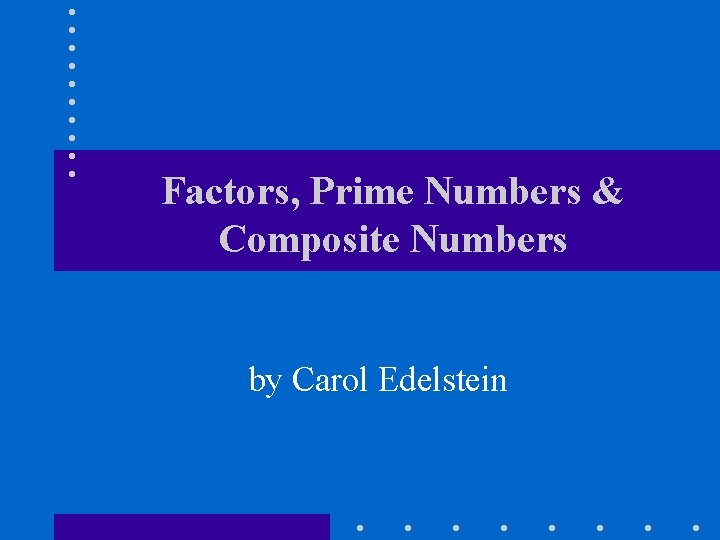Factors, Prime Numbers & Composite Numbers by Carol EdelsteinDefinition • Product – An answer to a multiplication problem. 7 x 8 = 56 Product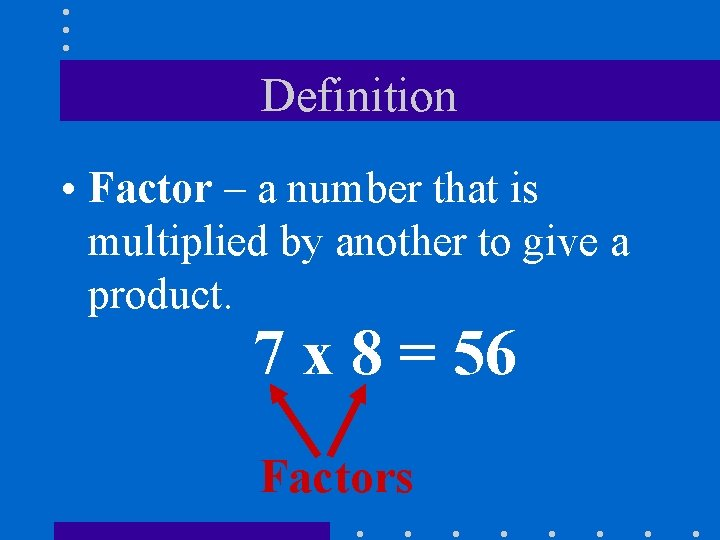Definition • Factor – a number that is multiplied by another to give a product. 7 x 8 = 56 FactorsDefinition • Factor – a number that divides evenly into another number. 56 ÷ 8 = 7 Factors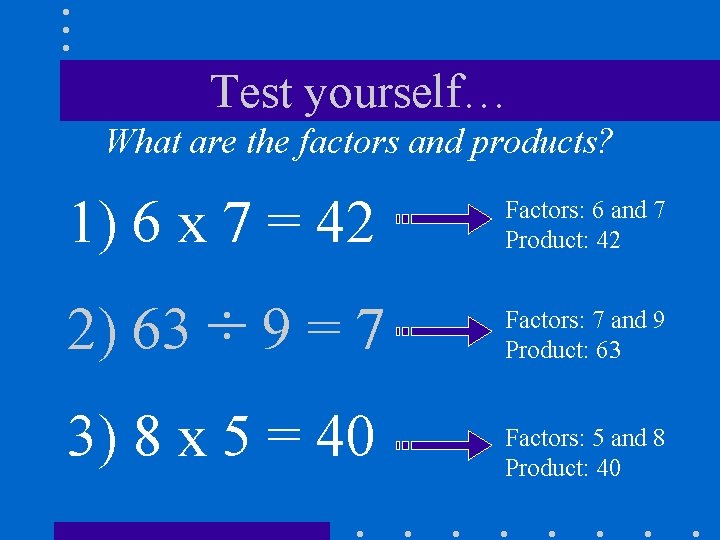Test yourself… What are the factors and products? 1) 6 x 7 = 42 Factors: 6 and 7 Product: 42 2) 63 ÷ 9 = 7 Factors: 7 and 9 Product: 63 3) 8 x 5 = 40 Factors: 5 and 8 Product: 40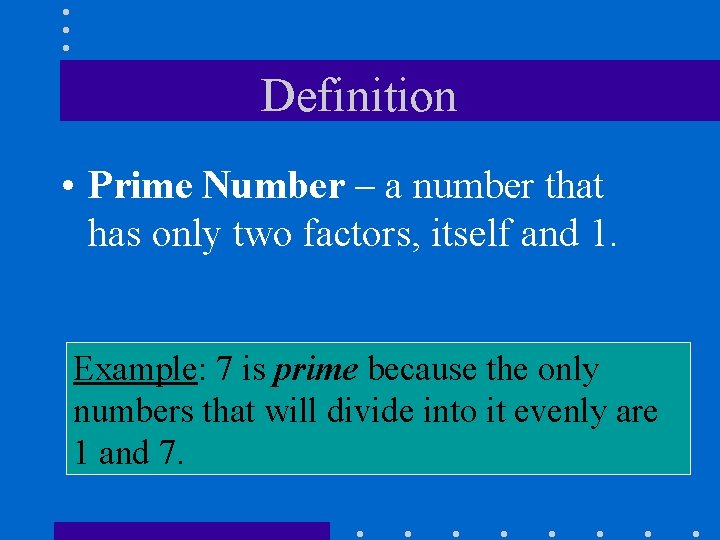Definition • Prime Number – a number that has only two factors, itself and 1. Example: 7 is prime because the only numbers that will divide into it evenly are 1 and 7.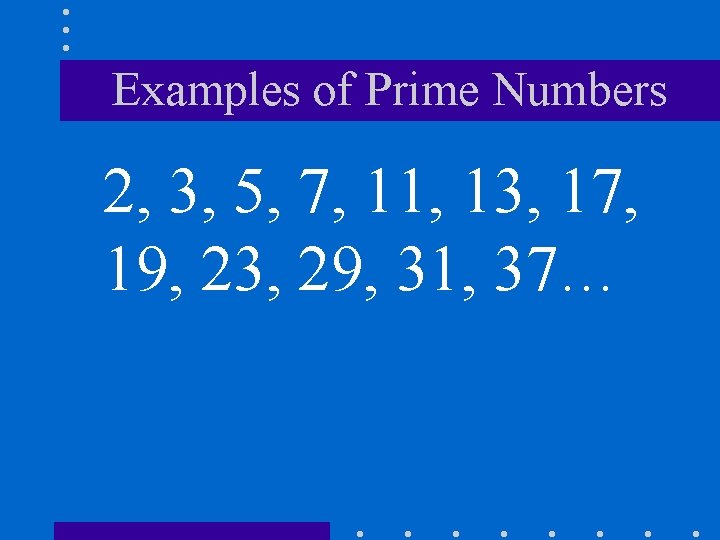Examples of Prime Numbers 2, 3, 5, 7, 11, 13, 17, 19, 23, 29, 31, 37…Definition • Composite number – a number that has more than two factors. Example: The number 8. The factors of 8 are 1, 2, 4, 8.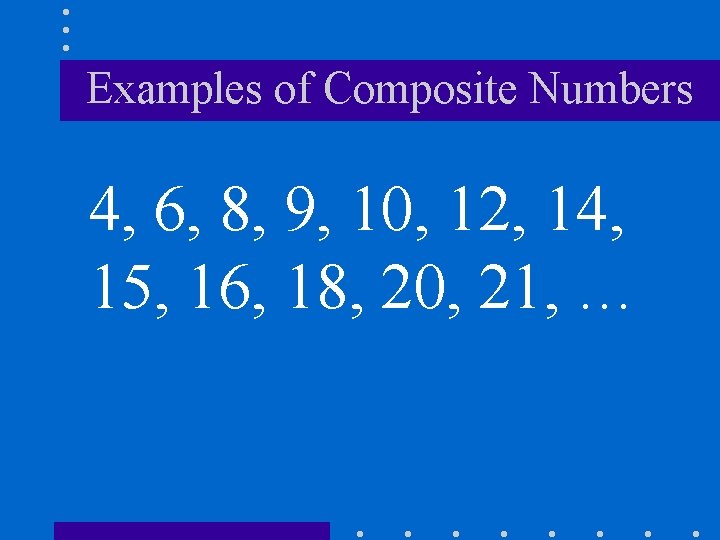Examples of Composite Numbers 4, 6, 8, 9, 10, 12, 14, 15, 16, 18, 20, 21, …One is special because. . . One is not prime. (because it does not have exactly two different factors). One is not Composite. (because it does not have more than 2 factors).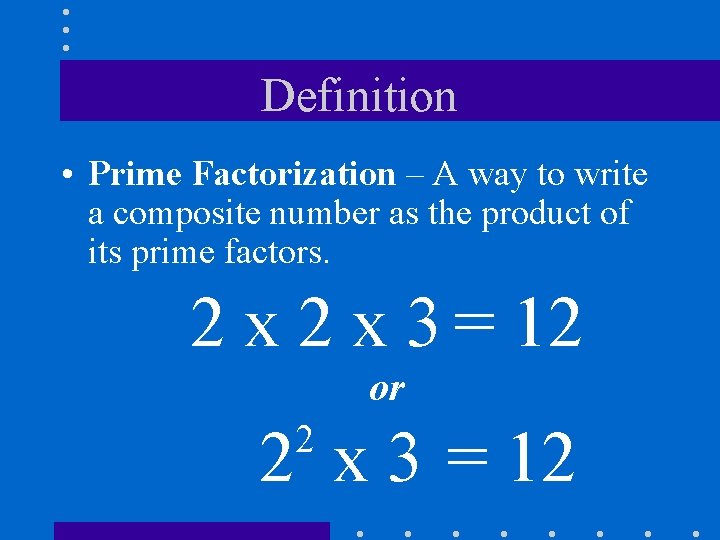Definition • Prime Factorization – A way to write a composite number as the product of its prime factors. 2 x 3 = 12 or 2 x 3 = 12 2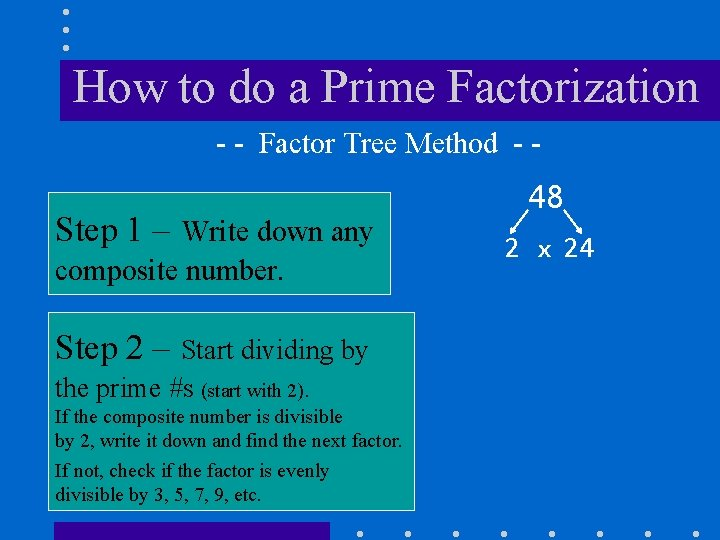How to do a Prime Factorization - - Factor Tree Method - - Step 1 – Write down any composite number. Step 2 – Start dividing by the prime #s (start with 2). If the composite number is divisible by 2, write it down and find the next factor. If not, check if the factor is evenly divisible by 3, 5, 7, 9, etc. 48 2 x 24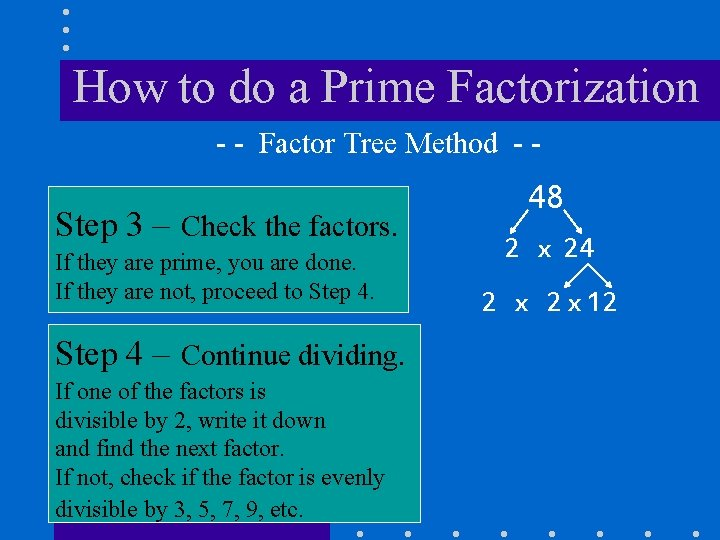How to do a Prime Factorization - - Factor Tree Method - - Step 3 – Check the factors. If they are prime, you are done. If they are not, proceed to Step 4 – Continue dividing. If one of the factors is divisible by 2, write it down and find the next factor. If not, check if the factor is evenly divisible by 3, 5, 7, 9, etc. 48 2 x 24 2 x 12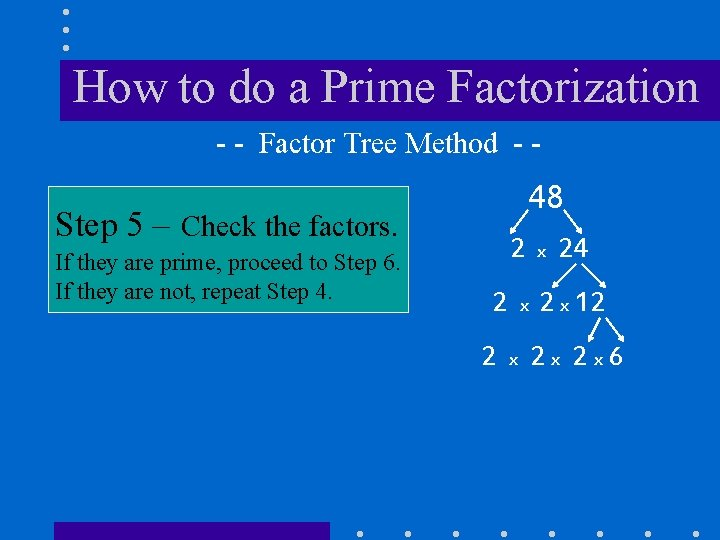How to do a Prime Factorization - - Factor Tree Method - - 48 Step 5 – Check the factors. If they are prime, proceed to Step 6. If they are not, repeat Step 4. 2 2 2 x x x 24 2 x 12 2 x 2 x 6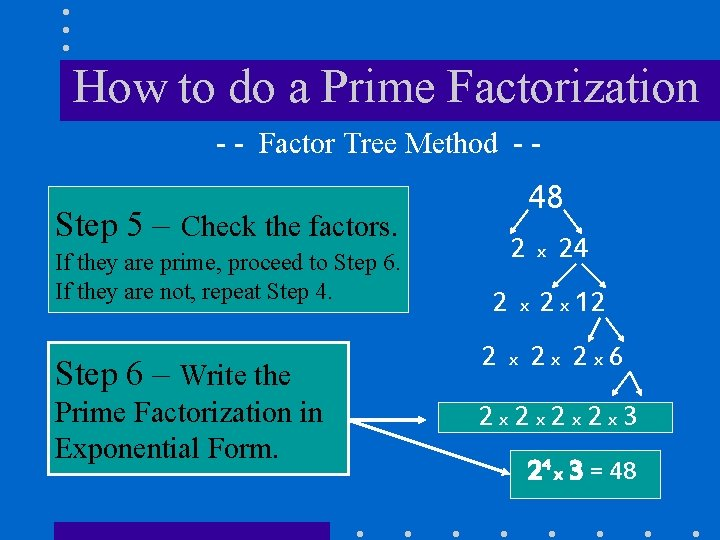How to do a Prime Factorization - - Factor Tree Method - - 48 Step 5 – Check the factors. If they are prime, proceed to Step 6. If they are not, repeat Step 4. 2 2 x x 24 2 x 12 Step 6 – Write the 2 Prime Factorization in Exponential Form. 2 x 2 x 3 x 2 x 2 x 6 24 x 3 = 48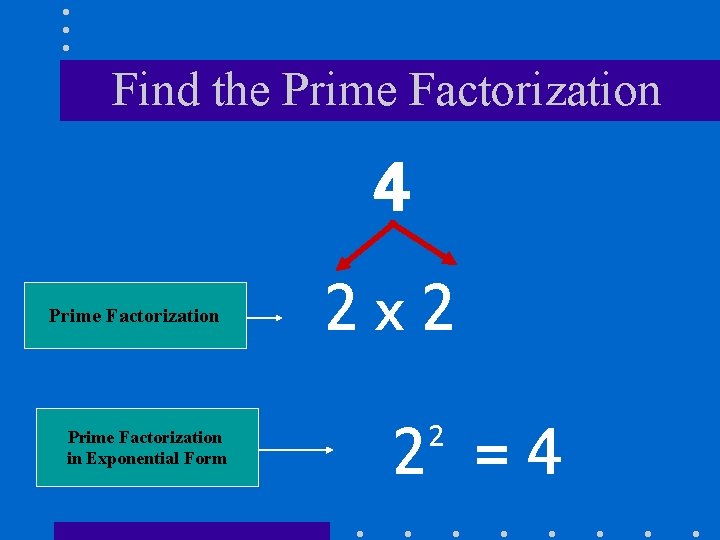Find the Prime Factorization 4 Prime Factorization in Exponential Form 2 x 2 2 =4 2Find the Prime Factorization 6 Prime Factorization 2 x 3 =6Find the Prime Factorization in Exponential Form 27 3 x 9 3 x 3 x 3 3 3 = 27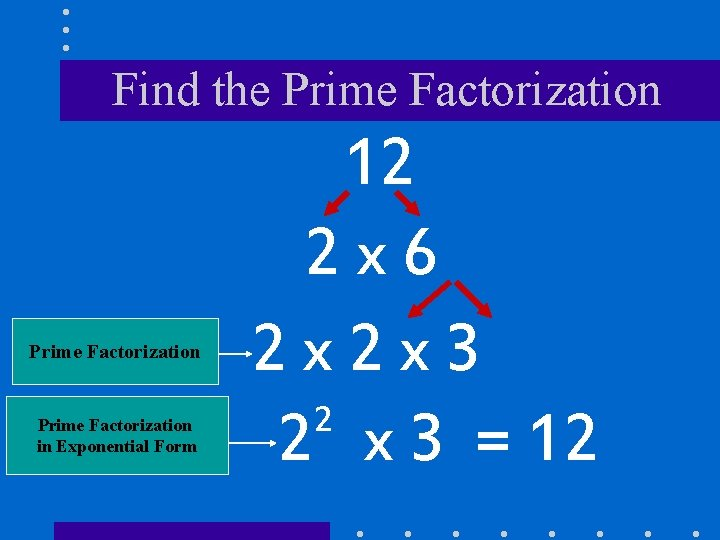Find the Prime Factorization in Exponential Form 12 2 x 6 2 x 2 x 3 2 2 x 3 = 12Find the Prime Factorization in Exponential Form 18 2 x 9 2 x 3 x 3 2 2 x 3 = 18You Have Options The following screens illustrate another method that you can use to find the Prime Factorization of a Composite Number. Try it! You may like it better.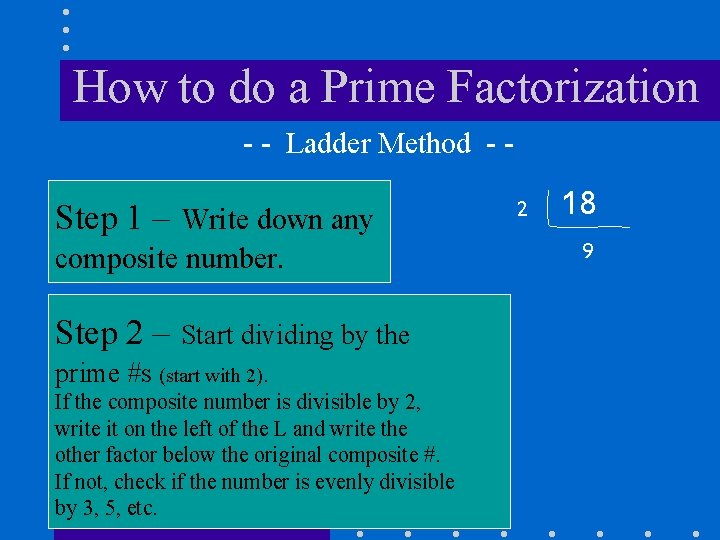How to do a Prime Factorization - - Ladder Method - - Step 1 – Write down any composite number. Step 2 – Start dividing by the prime #s (start with 2). If the composite number is divisible by 2, write it on the left of the L and write the other factor below the original composite #. If not, check if the number is evenly divisible by 3, 5, etc. 2 18 9How to do a Prime Factorization - - Ladder Method - - Step 3 – Check the factors. 2 18 If they are prime, proceed to Step 6. If not, continue the process. 3 9 Step 4 – Continue dividing the # on the next rung of the ladder by the prime #s (start with 2). Step 5 – Repeat this process until the # on the next rung of the ladder is prime. 3 Prime #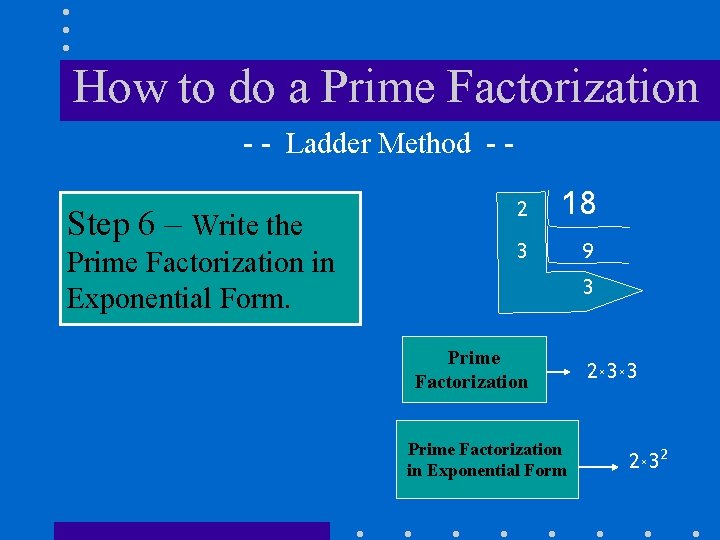How to do a Prime Factorization - - Ladder Method - - Step 6 – Write the Prime Factorization in Exponential Form. 2 18 3 9 3 Prime Factorization in Exponential Form 2 x 3 x 3 2 x 32Find the Prime Factorization - - Ladder Method - - Prime Factorization 2 2 56 2 14 7 28 222 7 x x x Prime # Prime Factorization in Exponential Form 23 x 7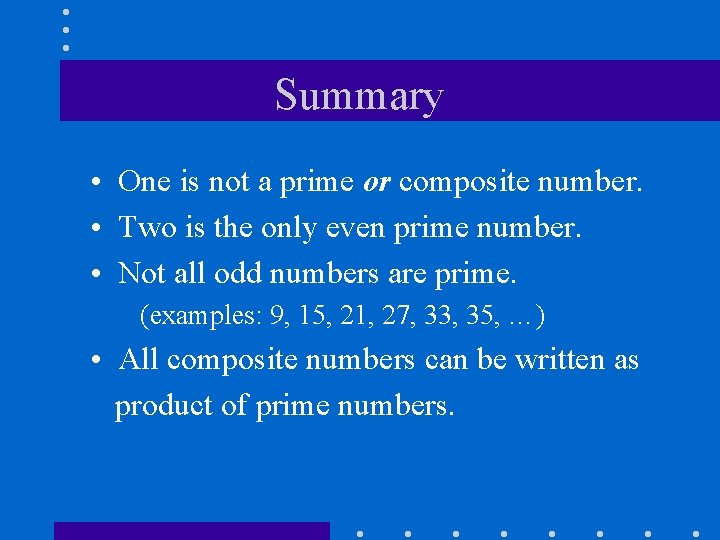Summary • One is not a prime or composite number. • Two is the only even prime number. • Not all odd numbers are prime. (examples: 9, 15, 21, 27, 33, 35, …) • All composite numbers can be written as product of prime numbers.The End